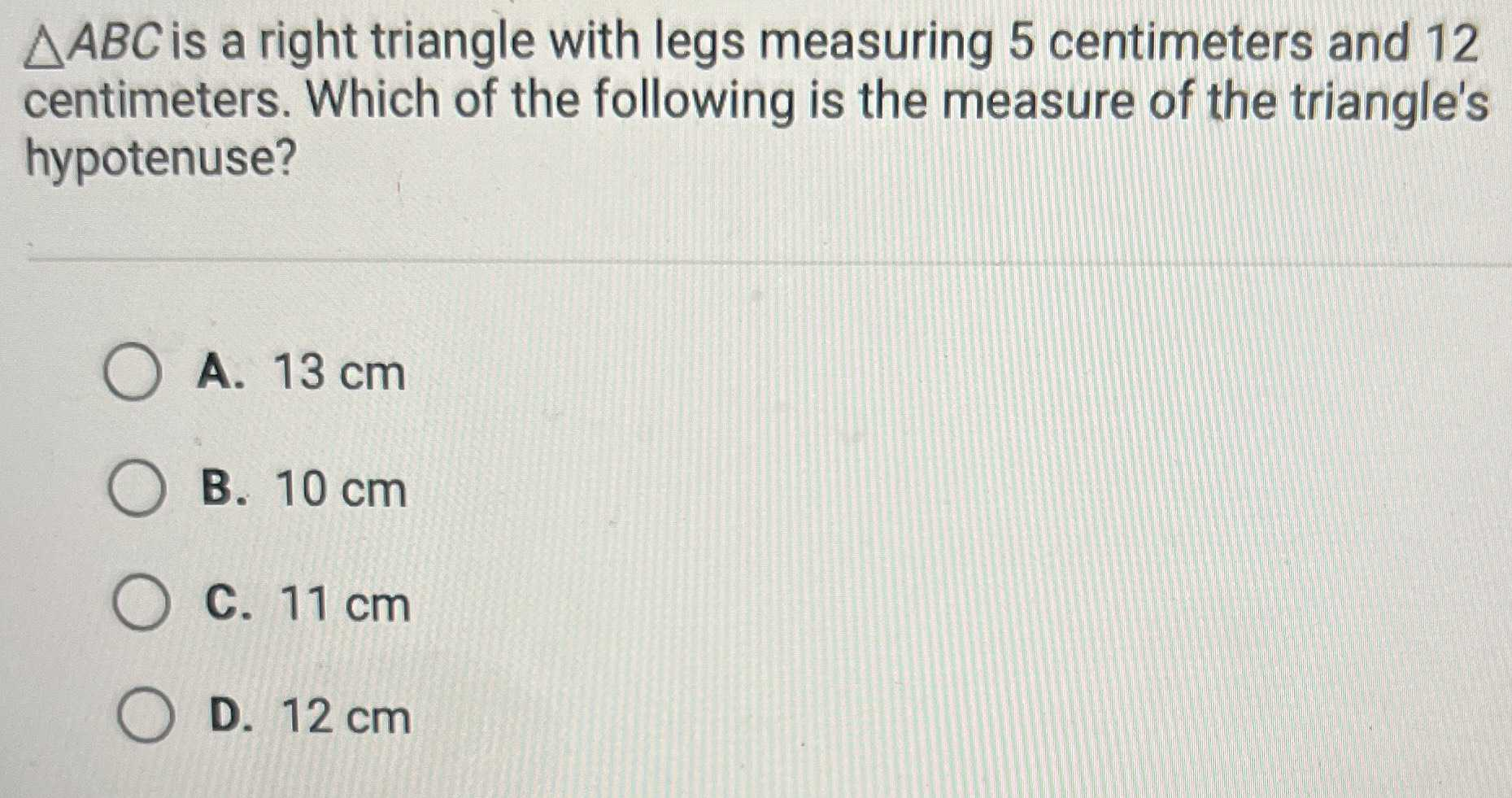### Still have math questions?

Trigonometry
Question$$\triangle A B C$$ is a right triangle with legs measuring $$5$$ centimeters and $$12$$ centimeters. Which of the following is the measure of the triangle's hypotenuse?

A. $$13 cm$$

B. $$10 cm$$

C. $$11 cm$$

D. $$12 cm$$